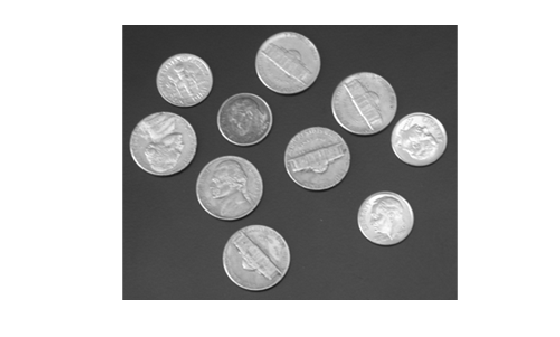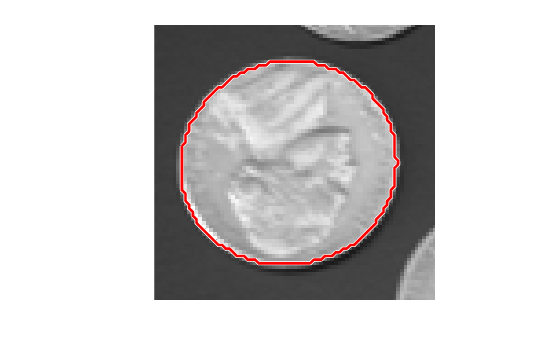Documentation

# reducepoly

Reduce density of points in ROI

## Syntax

``P_reduced = reducepoly(P)``
``P_reduced = reducepoly(P,tolerance)``

## Description

example

````P_reduced = reducepoly(P)` reduces the density of points in array `P`. Specify `P` as an n-by-2 array of the form ```[x1 y1; ...; xn yn]```, where each row represents a point. For example, `P` could be the array returned by one of the ROI creation functions, such as `drawfreehand` or `drawpolygon`. `reducepoly` returns `P_reduced`, an n-by-2 array of points that is typically smaller that `P`. `reducepoly` uses the Douglas-Peucker line simplification algorithm, removing points along a straight line and leaving only knickpoints (points where the line curves).```
````P_reduced = reducepoly(P,tolerance)` reduces the density of points in array `P`, where `tolerance` specifies how much a point can deviate from a straight line. Specify `tolerance` in the range `[0,1]`. ```

## Examples

collapse all

Read an image into the workspace.

```I = imread('coins.png'); ```

Convert the image from a grayscale image to a binary image. The function for finding boundaries requires binary images.

```bw = imbinarize(I); ```

Obtain the boundaries of all the coins in the binary image.

```[B,L] = bwboundaries(bw,'noholes'); ```

Plot the boundary for one of the coins over the original image.

```imshow(I) hold on; k = 1; boundary = B{k}; plot(boundary(:,2), boundary(:,1), 'r', 'LineWidth', 2) hold off; ```Use `reducepoly` to reduce the number of points defining the coin boundary.

```p = [boundary(:,2) boundary(:,1)]; tolerance = 0.02; % choose suitable tolerance p_reduced = reducepoly(p,tolerance); ```

Compare the original polygon overlaid over the reduced polygon and see how well the shape defined by fewer vertices matches the original polygon.

```hf = figure; ha = axes('parent',hf,'box','on','Ydir','reverse'); axis equal % Original data. line(p(:,1),p(:,2),'parent',ha,... 'color',[1 0.5 0],'linestyle','-','linewidth',1.5,... 'marker','o','markersize',4.5) % Reduced data. line(p_reduced(:,1),p_reduced(:,2),'parent',ha,... 'color',[0 0 1],'linestyle','-','linewidth',2,... 'marker','o','markersize',5); legend('Original points','Reduced points'); title('Douglas-Peucker algorithm'); ```## Input Arguments

collapse all

Points to be reduced, specified as an n-by-2 array of the form `[x1 y1; ...; xn yn]`. Each row in the array defines a vertex in a polyline, polygon, or freehand ROI shape.

Data Types: `single` | `double` | `int8` | `int16` | `int32` | `int64` | `uint8` | `uint16` | `uint32` | `uint64`

Sensitivity of the reduction algorithm, specified as a numeric scalar in the range `[0,1]`. Increasing the tolerance increases the number of points removed. A tolerance value of `0` has a minimum reduction in points. A tolerance value of `1` results in maximum reduction in points, leaving only the end points of the line.

Data Types: `single` | `double` | `int8` | `int16` | `int32` | `int64` | `uint8` | `uint16` | `uint32` | `uint64`

## Output Arguments

collapse all

Reduced data set, returned as a n-by-2 numeric array.

Data Types: `double`

## Algorithms

The Douglas-Peucker line simplification algorithm recursively subdivides a shape looking to replace a run of points with a straight line. The algorithm checks that no point in the run deviates from the straight line by more than the value specified by `tolerance`.